# 5G/NR - CSI RS Codebook                                       Home : www.sharetechnote.com

This page is about Beamforming of CSI RS. I think this would be the most complicated (tough to understand) part in NR protocol (at least to me). It will be helpful if you have the detailed understanding on LTE Beamforming (TM9) before you start reading on this topic.

Simply put, this is all about understanding 38.214-5.2.2.2 Precoding Matrix Indicator (PMI), but after reading this section several times I came to conclusion that it would be impossible for me to have complete understanding on this section without studying on additional documents outside of this specification (i.e, 38.214-5.2.2.2).

I am not sure how long it will take for me to reach to the certain level of understanding on this part but let's just start. This page will get updated by small steps for a long period as I learn more and get a little bit deeper understanding step by step.

Note : This is a topic with high degree of complexity, but this is not the whole story about CSI operation. It is only a small portions of the whole CSI framework. Once you have a certain level of understanding on this topic, I would suggest you to think of what role it plays in the whole CSI framework. See CSI Report and CSI RS page for further details on CSI framework operation.

What is Codebook ?

What is Codebook ? It would many different things in different situation, but the meaning of Codebook under the context of CSI-RS is a set of Precoders (a set of Precoding Matrix).

Do we(the reciever) should know the precoding matrix to decode the received signal ? The answer varies depending on how the data transmission scheme is designed. There are roughly two cases of implementation as shown below. In short, the reciever does not need to know of the precoding matrix information in Case 1 and the reciever need to know of the precoding matrix. (See Chapter 11 of Ref )

< Case 1 >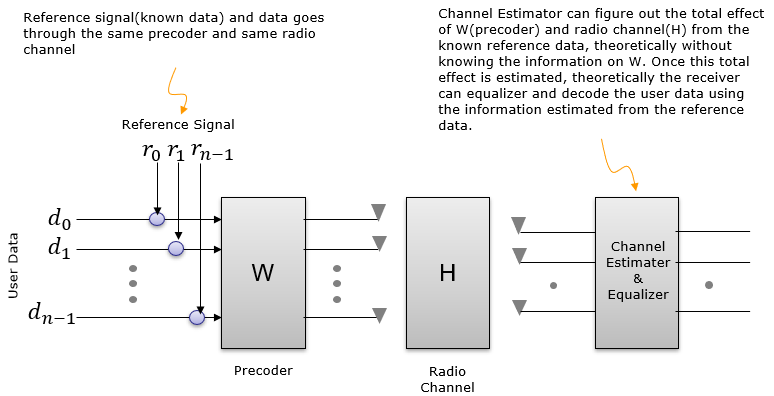< Case 2 >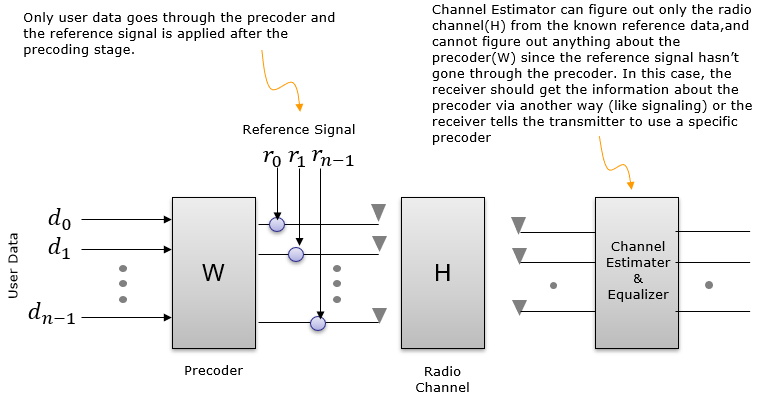NR implementation belong to < Case 1 >.

Most of LTE transmission Mode (e.g, TM1,2,3,4 etc) belong to < Case 2 >. That is, only data(e.g, PDSCH) is precoded and the reference signal for channel estimation (i.e, Cell Specific Reference Signal) is not precoded. In this case, UE should know of which precoding matrix is applied by eNB. UE knows of this based on predefined specification (as in TM1,2,3) or signaling information delivered by eNB(i.e, PMI index field in DCI).

But even in LTE, TM9 belong to < Case 1 > in which PDSCH carries its own demodulation reference signal. In this case, no precoding matrix is applied at the LTE precoding step. In stead, both PDSCH data and PDSCH DMRS would go through a beamforming process. This beamforming process can be regarded as a kind of precoding. Since both PDSCH data and PDSCH DMRS goes through the same beamforming process, UE does not need to know of the precoding matrix that eNB used.

Then why we care of the precoder (codebook) in NR. 'We don't need to know' doesn't mean 'it is useless'. Even if it is not mandatory, it will be definitely helpful for the reciever if it knows of the codebook.

Looking further into Reference Signal

Extending the explanation a little bit further into various possible cases with different type of reference signals, I classified them into following cases. The comments written here is what I had in my mind when I was drawing this diagram and the comments may not be very clear or even misleading if you go into details, but at least it helped me with understanding overal mathematical flow and refernce signal design in this way.

< Case 1 >

In this case, there is one reference signal called CRS. According to this diagram, this CRS is modified only by [H] matrix (Channel Matrix). If we assume that CRS is known to reciever in advance (e.g, by specification), the reciever can use this signal to estimate [H] by comparing the received CRS and the expected CRS (the CRS specified in specification). Then the question is 'can the reciever decode User data(d) only with knowledge of [H] ?'. Highly likely NOT because the user data is modified by not only by [H] but also by [W]. Then next question is 'can the reciever estimate (figure out) [W] ? The reciever cannot know [W] directly from the recieved signal. The information about [W] should be known to the reciever by other means like signaling message or physical control information or predefined specification.

LTE using Cell specific Reference Signal belong to this case.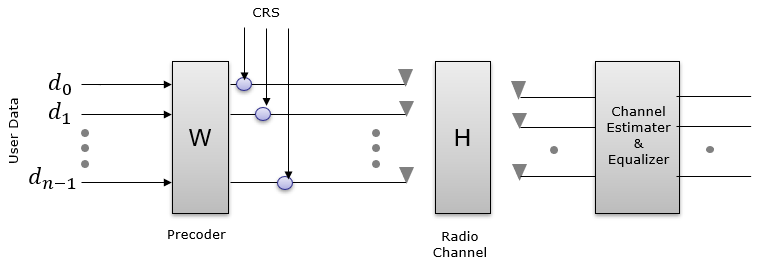< Case 2 >

In this case, there is one reference signal called DMRS. According to this diagram, this DMRS is modified only by [W](Precoding matrix) and [H] matrix (Channel Matrix). If we assume that DMRS is known to reciever in advance (e.g, by specification), the reciever may be able to decode user data by correcting the received signal with DMRS. But if you ask 'Can UE estimate [W] and [H] ?'. Highly likely No. Reciever can estimate the product of [H][W] by comparing the received DMRS and the expected DMRS, but it would not be possible to estimate [H] and [W] separately.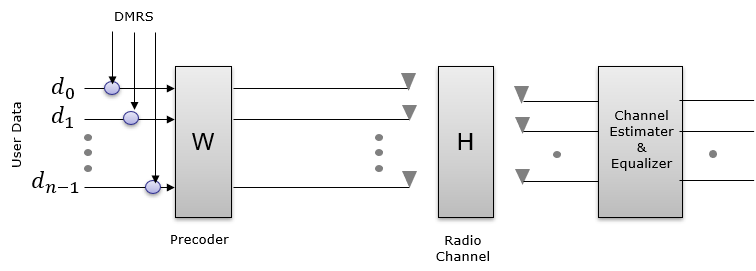< Case 3 >

In this case, there is two reference signal called DMRS and CSI-RS. According to this diagram, this DMRS is modified only by both [W](Precoding matrix) & [H] matrix (Channel Matrix) and CSI-RS is modified by [H]. The reciever can estimate [H] matrix, but W matrix may not be directly estimated from DMRS since DMRS is modified by both [W] and [H] so [W] information would be given to the reciever by signaling or predefined specification. (Theoretically, if the reciver using both the [H] estimated from CSI-RS and DMRS, it may estimate [W]). In theory, in this case the reciever would be able to decode User Data using DMRS only. If CSI-RS would be helpful additional information, but it would not be critical.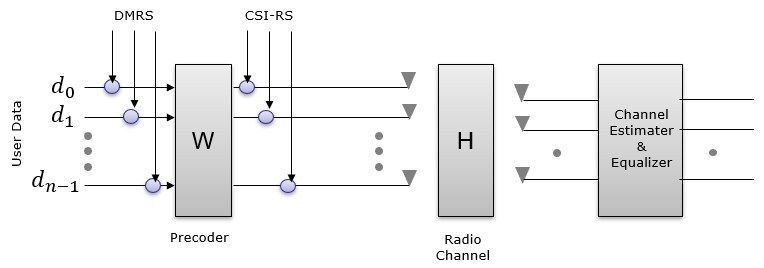< Case 4 >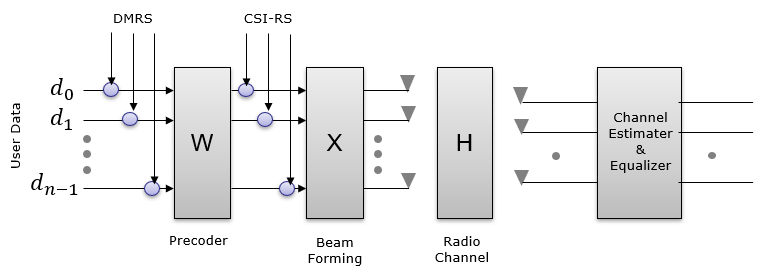How can UE or gNB knows about the precoder information ?

In UE's perspective, a typical way is to send measurement report for PMI to gNB and may assume that gNB would use the PMI(Precoding Matrix Indicator) that it reports... but the important thing to remember is ... it is not mandatory for gNB to use the PMI that UE report. It may or may not use the same PMI depending on its own situation. Even if gNB use different PMI than what UE report, UE can decode the downlink data because the downlink signal is processed as < Case 1 > in previous section.

In gNB's perspective, a typical way is to tell UE to send SRS and pick a specific PMI based the channel estimation of SRS, and tell UE via DCI 0_1 (TMPI field) to use a specific PMI. In this case, UE shall use the specific precoding matrix specified by gNB.

The first problem that I got when I start reading the section 38.214-5.2.2.2 was to figure out the meaning of strange looking single character parameters like N1,N2,O1,O2 which is not clearly defined in the TS(Technical Specification) and some other terminology like 'Panel' (as in single panel, multi panel as shown in the RRC message). When some terminolgy is not clearly defined in TS document, it is highly likely that they are described in some TDocs (R1-XXXXX) and transferred to TS document without clear explanation. So I started search TDocs about this topic and I found a TDoc Ref ,,,, which seems to be the origin of this topic and some other documents as in Ref  and Ref  that would help us to understand other terminology like 'Panel'.

NOTE : Basically, most of the fundamental concept of NR CSI Codebook design is inherited from LTE Rel 13 FD-MIMO codebook design. You would find a lot of detailed description and diagrams in LTE TDocs. Check in the link in Ref  , Ref and take a look into the documents on CSI Coddebook.

N1,N2,O1,O2 : Definition of these single letter parameters are illustrated in R1-1612661 as shown below. N1,N2 is determined by the number of antenna in horizontal and vertical direction. O1, O2 indicates DFT Oversampling.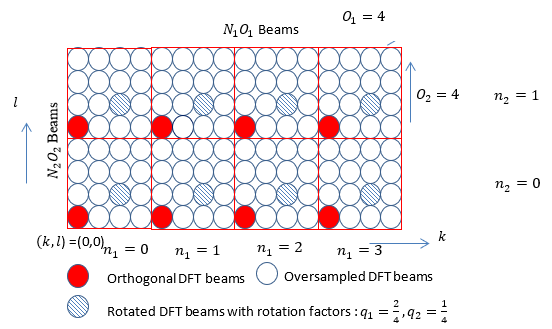N1, N2, O1, O2 are defined in 3GPP as shown below. I've added some additional column to the table since these additional columns are used often in other parts.

<Type 1 Single Panel : Based on 38.214 v15.3-Table 5.2.2.2.1-2: Supported configurations of (N1,N2) and (O1,O2)>

 Number of CSI-RS antenna ports (N1,N2) 2*N1*N2 = # of CSI ports (O1,O2) N1*O1 N2*O2 N1*O1*N2*O2=Ac (Bitmap in SinglePanel n1-n2) 4 (2,1) 2*2*1 = 4 (4,1) 2*4=8 1*1=1 2*4*1*1 = 8 8 (2,2) 2*2*2 = 8 (4,4) 2*4=8 2*4=8 2*4*2*4 = 64 (4,1) 2*4*1 = 8 (4,1) 4*4=16 1*1=1 4*4*1*1 = 16 12 (3,2) 2*3*2 = 12 (4,4) 3*4=12 2*4=8 3*4*2*4 = 96 (6,1) 2*6*1 = 12 (4,1) 6*4=24 1*1=1 6*4*1*1 = 24 16 (4,2) 2*4*2 = 16 (4,4) 4*4=16 2*4=8 4*4*2*4 = 128 (8,1) 2*8*1 = 16 (4,1) 8*4=32 1*1=1 8*4*1*1 = 32 24 (4,3) 2*4*3 = 24 (4,4) 4*4=16 3*4=12 4*4*3*4 = 192 (6,2) 2*6*2 = 24 (4,4) 6*4=24 2*4=8 6*4*2*4 = 192 (12,1) 2*12*1 = 24 (4,1) 12*4=48 1*1=1 12*4*1*1 = 48 32 (4,4) 2*4*4 = 32 (4,4) 4*4=16 4*4=16 4*4*4*4 = 256 (8,2) 2*8*2 = 32 (4,4) 8*4=32 2*4=8 8*4*2*4 = 256 (16,1) 2*16*1 = 32 (4,1) 16*4=64 1*1=1 16*4*1*1 = 64

NOTE : It is noted that O1 is always 4 and O2 has only two possibilities : 1 or 4.

<Type 1 Multi Panel : Based on 38.214 v15.3-Table 5.2.2.2.2-1: Supported configurations of (Ng,N1,N2) and (O1,O2)>

 Number of CSI-RS antenna ports (Ng,N1,N2) 2*Ng*N1*N2 = # of CSI ports (O1,O2) N1*O1 N2*O2 N1*O1*N2*O2=Ac (Bitmap in MultiPanel ng-n1-n2) 8 (2,2,1) 2*2*2*1 = 8 (4,1) 2*4=8 1*1=1 2*4*1*1 = 8 16 (2,4,1) 2*2*4*1 = 16 (4,1) 4*4=16 1*1=1 4*4*1*1 = 16 (4,2.1) 2*4*2*1 = 16 (4,1) 2*4=8 1*1=1 1*4*1*1 = 8 (2,2,2) 2*2*2*2 = 16 (4,4) 2*4=8 2*4=8 2*4*2*4 = 64 32 (2,8,1) 2*2*8*1 = 32 (4,1) 8*4=32 1*1=1 8*4*1*1 = 32 (4,4,1) 2*4*4*1 = 32 (4,1) 4*4=16 1*1=1 4*4*1*1 = 16 (2,4,2) 2*2*4*2 = 32 (4,4) 4*4=16 2*4=8 4*4*2*4 = 128 (4,2,2) 2*4*2*2 = 32 (4,4) 2*4=8 2*4=8 2*4*2*4 = 64

NOTE : It is noted that O1 is always 4 and O2 has only two possibilities : 1 or 4.

Panel : The definition of the panel can be illustrated as below (I don't think I need explain further in writing). 3GPP support both Single Panel and Multi Panel. In terms of Multi Panel, the current 3GPP (TS 38.214 / 38.331) seems to support only Multi-Panel Uniform case.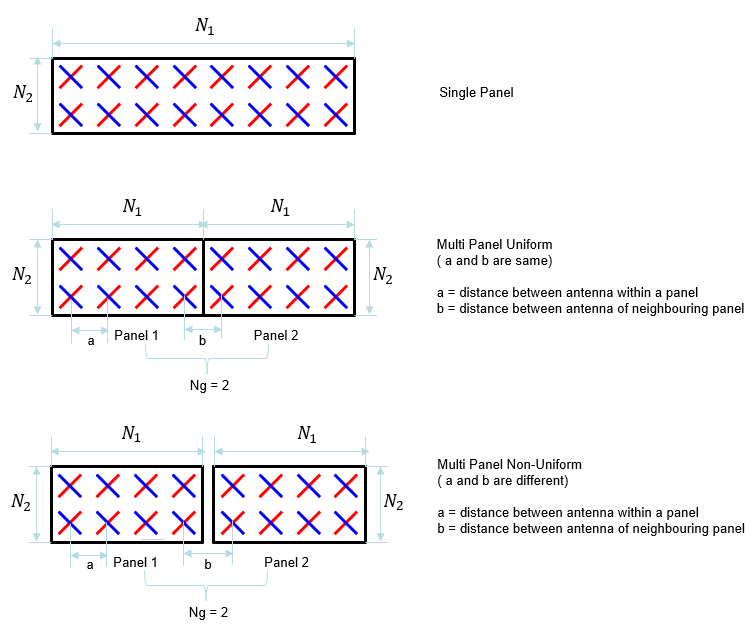Using this definition, we can visualize the antenna array for each codebook as follows. As you see here, you would notice that there are multiple options of array structure for the same number of CSI-RS antenna ports.  Why they propose multiple options ? that is, what is the difference between different options. For example, what would be the difference between 4x1 array and 2x2 array with 8 CSI port ?  The main difference caused by this different structure is the shape of the beam in 3D space. For more intuitive understanding, I put another type of my notes in www.slide4math.com. Check out [Array Antenna] menu in www.slide4math.com.

<Type 1 Single Panel>

 Number of CSI-RS antenna ports (N1,N2) CSI Antenna Port Array (Logical Configuration) 4 (2,1)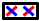8 (2,2)(4,1)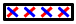12 (3,2)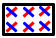(6,1)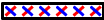16 (4,2)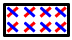(8,1)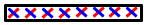24 (4,3)(6,2)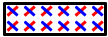(12,1)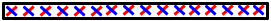32 (4,4)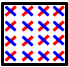(8,2)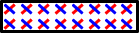(16,1)<Type 1 Multi Panel>

 Number of CSI-RS antenna ports (Ng,N1,N2) CSI Antenna Port Array (Logical Configuration) 8 (2,2,1)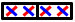16 (2,4,1)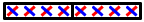(4,2.1)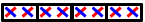(2,2,2)32 (2,8,1)(4,4,1)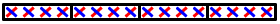(2,4,2)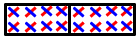(4,2,2)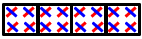As mentioned above, it is very diffuclt to understand the specification about CSI-RS codebook just by reading 3GPP TS specification. So in my case I often look into various TDocs and sometimes textbooks about 5G Physical layer. However, in many case it is hard to find direct connection between what TDoc/Textbook says and what 3GPP TS says. In this section, I would put some tips and hints on how to correlate what you usually see from TDoc/Textbook with what 3GPP TS specifies.

The first thing I want to show you is the link shown below. In most TDocs and Textbook, they describe CSI Codebook as W = W1 * W2 (the combination of two matrices) but 3GPP TS does not explicitely describes it in the same way. 3GPP TS just list a whole bunch of tables. The connection between the Codebook in TDoc and 3GPP TS can be illustrated as follows.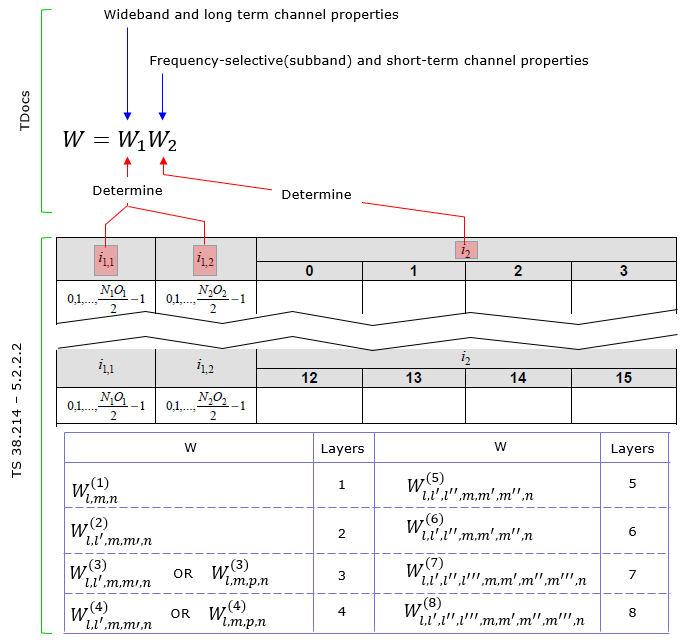Focusing further into the codebook matrix format, 3GPP TS defines various different form of W matrix depending on the number of layers and some other parameters as shown below.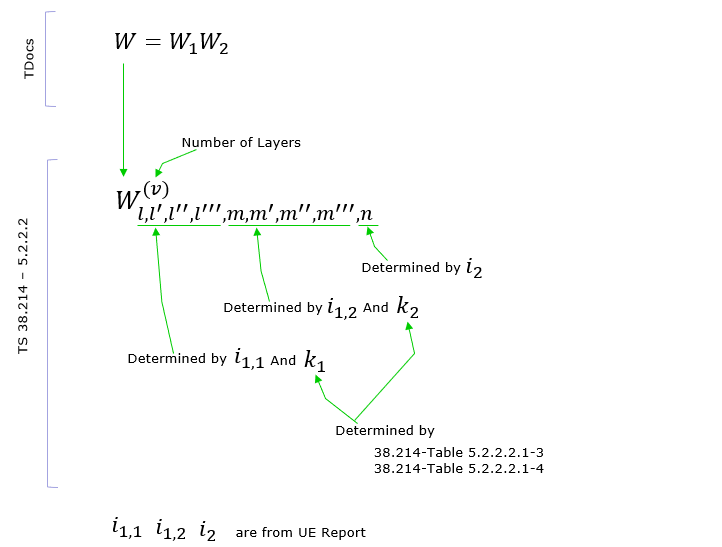NOTE : Regarding the derivation of W matrix and the meaning of each elements of the matrix, I would suggest you to refer to Ref . It is mostly for LTE CSI Codebook design, but the basic concept of NR CSI Codebook design (especially Type 1) is inherited from LTE CSI Codebook design.

As you may notice from what is mentioned above, there are several parameters labeled as 'i something' like i1_1, i1_2, i_2. According to what I mentioned above, you would figure out that i1_x (i.e, i1_1,i1_2 etc) determines wideband properties and i2 determines subband properies. This relationship specified in 38214-5.2.2.2 as below (In my note, i1_3 is not explicitely described. I would mention on this when I have chance to explain on each specific CSI codebook table in the future. For now, just let you know that i1_3 is an additional parameter that are required to determine the codebook for multi layer).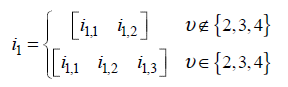The construction process of W1 matrix is illustrated as below. As you see, this matrix is associated with antenna structure. Each colum of this matrix represents the weight vector for each specific beam formed by the antenna array. (NOTE : In some documents, the vector X1, X2 is described as B).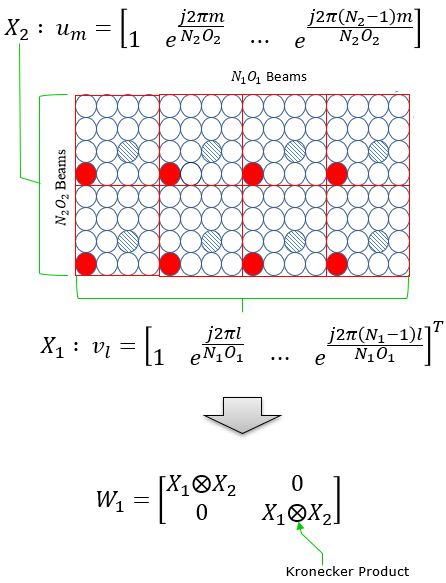W2 is in the form of vector that functions to select specific beams (columns) of W1.

Even though most of TDocs and Textbook explain about Precoding Matrix using two matrix W1 and W2 , you may not recognize clearly separated matrix in the W matrix given in 3GPP PMI tables. So I would like to give you overall structure of the W matrix given in 3GPP PMI tables. You would see roughly two types of W Matrix structure as below depending on the PMI table you pick.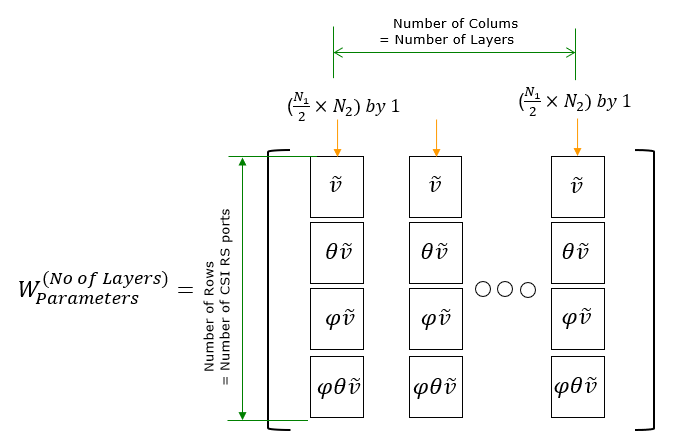With this general structure in mind, pick a very simple PMI table that you think you can handle and derive the matrix with pen and pencil as I did in Derivation of W matrix Example. There might be some mistakes that I made in this derivation, but at least it helped me to build much clearer picture on the structure and meaning of the matrix.

Note : Through this note, (I hope) you would get pretty concrete understanding on how a precoding matrix is derived for a specific CSI reference setting. Once you manage to derive such a matrix, then you would want to understand how to interpret the matrix (i.e, how the matrix is applied to the data to be transmitted). For intuitive understanding on those matrix, I would suggest to make practice on how a complex matrix transform a set of complex data(I/Q data). For this type of practice, I posted another style of my notes at www.slide4math.com. See [Engineering]->->[Matrix Complex].

As explained in previous section, CSI report in NR is made up of two categories(granularities). One is for Wideband property (Wideband Granularity) and the other one is subband property(subband granularity). These two parameters can be set separately and reported in independent scheduling, which gives more efficiency and flexibility for CSI framework (it may make it difficult for us to understand the mechanism though).

According to 38.214 - 5.2.1.4, Wideband and Subband Report properties can be configured by ReportQuantity in RRC message as follows :

A CSI Reporting Setting is said to have a wideband frequency-granularity if

• reportQuantity is set to 'cri-RI-PMI-CQI', or 'cri-RI-LI-PMI-CQI', cqi-FormatIndicator indicates single CQI
• reporting and pmi-FormatIndicator indicates single PMI reporting, or
• reportQuantity is set to 'cri-RI-i1' or
• reportQuantity is set to 'cri-RI-CQI' or 'cri-RI-i1-CQI' and cqi-FormatIndicator indicates single CQI reporting, or
• reportQuantity is set to 'cri-RSRP' or 'ssb-Index-RSRP'

otherwise, the CSI Reporting Setting is said to have a subband frequency-granularity.

PMI Tables

Followings are summaries on correlations on Type, number of layers, number of CSI antenna ports & dimension and specific mapping table. But it would not be easy to make sense out of this summary table. To understand each of these tables in detail, I would suggest you go through TDocs in reference section (or at least high level descriptions in this note) to get some big picture first and then you need to construct a few specific PMI matrix by hands as I did in example sections.

 Type # of Layers Antenna Port Codebook Mode Pcsi-RS N2 Ng Table in 38.214 Type I Single-Panel 1 3000~3001 Table 5.2.2.2.1-1 2 3000~3001 Table 5.2.2.2.1-1 1 3000~ 2999 + Pcsi-RS 1 Table 5.2.2.2.1-5 1 3000~ 2999 + Pcsi-RS 2 > 1 Table 5.2.2.2.1-5 1 3000~ 2999 + Pcsi-RS 2 1 Table 5.2.2.2.1-5 2 3000~ 2999 + Pcsi-RS 1 Table 5.2.2.2.1-6 2 3000~ 2999 + Pcsi-RS 2 > 1 Table 5.2.2.2.1-6 2 3000~ 2999 + Pcsi-RS 2 1 Table 5.2.2.2.1-6 3 3000~ 2999 + Pcsi-RS 1-2 < 16 Table 5.2.2.2.1-7 3 3000~ 2999 + Pcsi-RS 1-2 >= 16 Table 5.2.2.2.1-7 4 3000~ 2999 + Pcsi-RS 1-2 < 16 Table 5.2.2.2.1-8 4 3000~ 2999 + Pcsi-RS 1-2 >= 16 Table 5.2.2.2.1-8 5 3000~ 2999 + Pcsi-RS 1-2 Table 5.2.2.2.1-9 6 3000~ 2999 + Pcsi-RS 1-2 Table 5.2.2.2.1-10 7 3000~ 2999 + Pcsi-RS 1-2 Table 5.2.2.2.1-11 8 3000~ 2999 + Pcsi-RS 1-2 Table 5.2.2.2.1-12 Type I Multi Panel 1 3000~ 2999 + Pcsi-RS 1 {2,4} Table 5.2.2.2.2-3 1 3000~ 2999 + Pcsi-RS 2 2 Table 5.2.2.2.2-3 2 3000~ 2999 + Pcsi-RS 1 {2,4} Table 5.2.2.2.2-4 2 3000~ 2999 + Pcsi-RS 2 2 Table 5.2.2.2.2-4 3 3000~ 2999 + Pcsi-RS 1 {2,4} Table 5.2.2.2.2-5 3 3000~ 2999 + Pcsi-RS 2 2 Table 5.2.2.2.2-5 4 3000~ 2999 + Pcsi-RS 1 {2,4} Table 5.2.2.2.2-6 4 3000~ 2999 + Pcsi-RS 2 2 Table 5.2.2.2.2-6 Type II 1 & 2 3000~ 2999 + Pcsi-RS Table 5.2.2.2.3-5 Type II Port Selection 1 & 2 3000~ 2999 + Pcsi-RS Table 5.2.2.2.4-1

< Table 5.2.2.2.1-1: Codebooks for 1-layer and 2-layer CSI reporting using antenna ports 3000 to 3001 >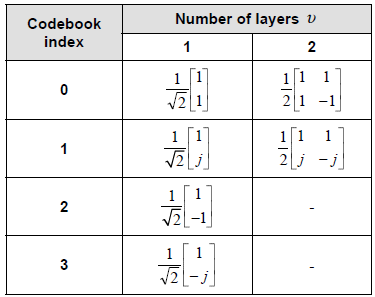< Table 5.2.2.2.1-5: Codebook for 1-layer CSI reporting using antenna ports 3000 to 2999+P_CSI-RS >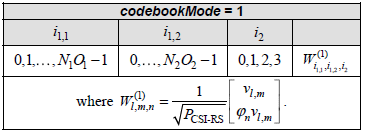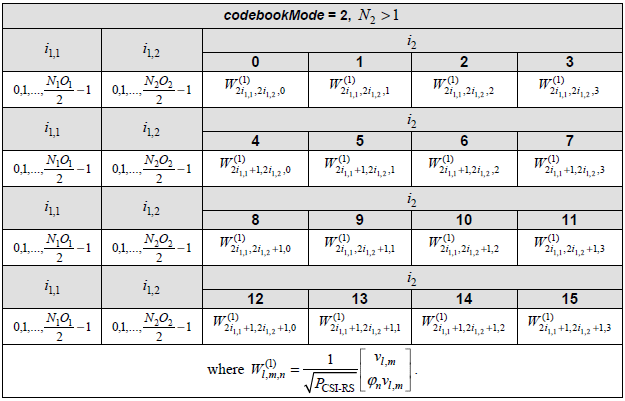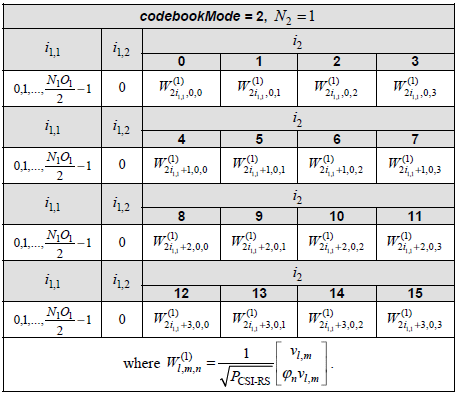< Table 5.2.2.2.1-6: Codebook for 2-layer CSI reporting using antenna ports 3000 to 2999+P_CSI-RS >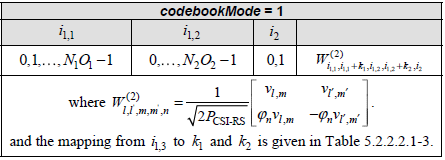[ Table 5.2.2.2.1-3: Mapping of i1,3 to k1 and k2 for 2-layer CSI reporting ]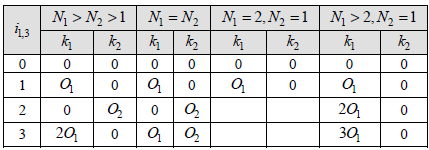[ Table 5.2.2.2.1-3: Mapping of i1,3 to k1 and k2 for 2-layer CSI reporting ][ Table 5.2.2.2.1-3: Mapping of i1,3 to k1 and k2 for 2-layer CSI reporting ]In this section, the final matrix you will derive will be in the form of complex number elements. The user data (i.e, PDSCH data) is also in the form of complex number (I and Q data). Eventually you should be able to visualize the properties of these matrix in your brain to make sense out of those matrix. Here goes another type of my notes that may help you with the intuitive understandings on the matrix with complex number elements being applied to complex data. Play with [Matrix Complex] in www.slide4math.com.

Example 01 >

Let's assume that PMI table is configured according to following table.Suppose we have following report and antenna configuration as follows :

No of CSI ports = 4

N1 = 2, N2 = 1, O1 = 4, O2 = 1

i1,1 = 0  i1,2 = 0  i2 = 0. This indicates l = i1,1 = 0, m = i1,2 = 0 , n = i2 = 0

Now, let's plug these parameters into all the equations that lead to final matrix. I hope I did all the steps right. Let me know if you find anything wrong.

First, figure out um vector.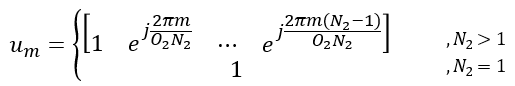In this example, N2 is 1. So um becomes as follows.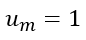Next, figure out vl,m vector.In this example, l = 0, m = 0. so vl,m become as follows :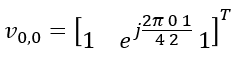Transposing the vector, we get following vector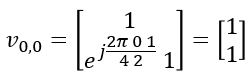Now calculaing Phi value using the parameter n.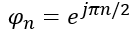Plugging into n value given in this example, we get the value as follows.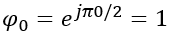Now plugging into the matrix all the vectors we got abovePlugging l,m,n value given in this example, we get following vector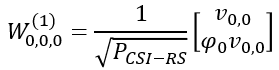Do all the calculation to the end, we get the final matrix(vector) as follows.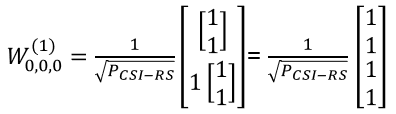Example 02 >

Let's assume that PMI table is configured according to following table.Suppose we have following report and antenna configuration as follows :

No of CSI ports = 4

N1 = 2, N2 = 1, O1 = 4, O2 = 1

i1,1 = 1  i1,2 = 0  i2 = 1. This indicates l = i1,1 = 1, m = i1,2 = 0 , n = i2 = 1

Now, let's plug these parameters into all the equations that lead to final matrix. I hope I did all the steps right. Let me know if you find anything wrong.

First, figure out um vector.In this example, N2 is 1. So um becomes as follows.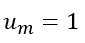Next, figure out vl,m vector.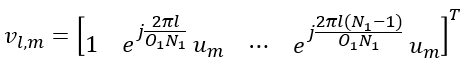In this example, l = 0, m = 0. so vl,m become as follows :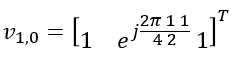Transposing the vector, we get following vector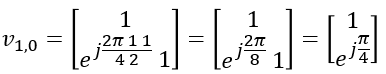Now calculaing Phi value using the parameter n.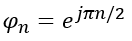Plugging into n value given in this example, we get the value as follows.Now plugging into the matrix all the vectors we got abovePlugging l,m,n value given in this example, we get following vector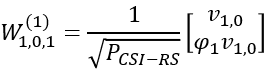Do all the calculation to the end, we get the final matrix(vector) as follows.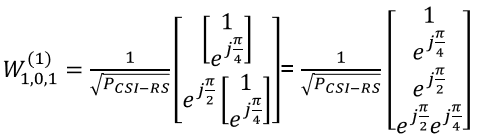Note : Now the last step is to understand the meaning of this vector. The best way would be that you yourself create a bunch of IQ data and multiply this vector with the IQ data and see how the original IQ data changes. The second best way (in case you don't want to do this on your own) would be to take a look at my visual math note. See this page at [Engineering]->->[Matrix Complex] in www.slide4math.com . Also you can interpret this matrix as a beam as shown in this page.

Example 03 >

Let's assume that PMI table is configured according to following table.Suppose we have following report and antenna configuration as follows :

No of CSI ports = 4

N1 = 2, N2 = 1, O1 = 4, O2 = 1

i1,1 = 0,  i1,2 = 0,  i1,3 = 0, i2= 0.

This indicates

k1 = 0, k2 = 0

l = i1,1 = 0, l�= i1,1+k1=0,  m = i1,2 = 0, m� =  i1,2+k2 = 0, n = i2 = 0

Let's figure out um vector first.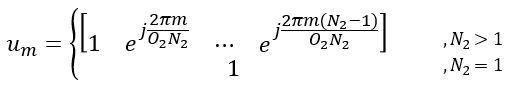Since N2 = 1 in the given condition in this example, um become 1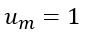Now let's derive vl,m vector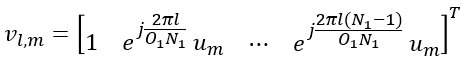Since both l and m is 0 in this example, the vector become as follows.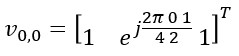Transposing the vector and simplifing it, we get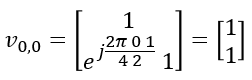Calculating Phi with n given in this example, we get the phi value as follows.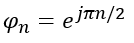In the same as we did for vl,m, we can calculate vl',m' from following equation.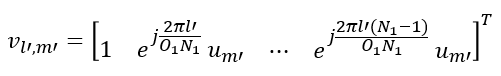Plugging the number for l' and m', we get following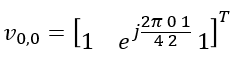Transposing and Simplifying, we get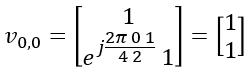Now let's plug into the final matrix all the vectors and values that we derived above.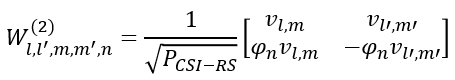Rewriting the matrix by plugging in parameters (l,l',m,m',n), it looks as follows.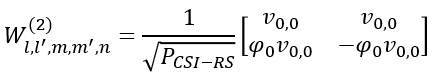Then, plugging the vectors and values derived above into the matrix, we get the final result as follows.Example 04 >

Let's assume that PMI table is configured according to following table.Suppose we have following report and antenna configuration as follows :

No of CSI ports = 4

N1 = 2, N2 = 1, O1 = 4, O2 = 1

i1,1 = 1,  i1,2 = 0,  i1,3 = 1, i2= 1.

This indicates

k1 = O1=4, k2 =0 [Note : Since this is the case where N1 > N2 > 1, k1 becomes O1 and k2 becomes 0 when i1,3 = 1]

l = i1,1 = 1, l�= i1,1+k1=1+4=5,  m = i1,2 = 0, m� =  i1,2+k2 = 0+0 = 0, n = i2 = 1

Let's figure out um vector first.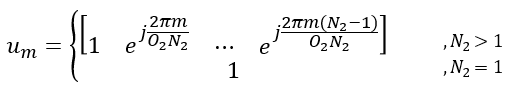Since N2 = 1 in the given condition in this example, um become 1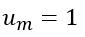Now let's derive vl,m vector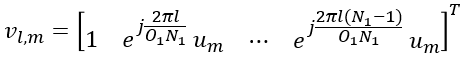Since both l is 1 and m is 0 in this example, the vector become as follows.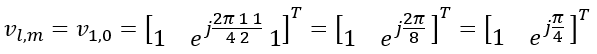Transposing the vector and simplifing it, we getCalculating Phi with n given in this example, we get the phi value as follows.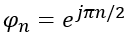Now let's derive vl',m' from the following vector.Plugging l' and m' into the equation, we get a vector as follows.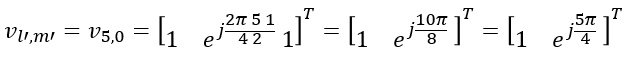Trransposing and Symplifying the vector, we get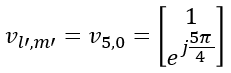Now let's derive the final matrix from following equation.Plugging the vectors and parameters we got in previous step , we get following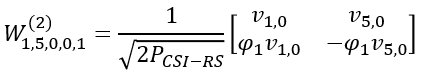Plugging the number and simplifying, we get the final result as follows :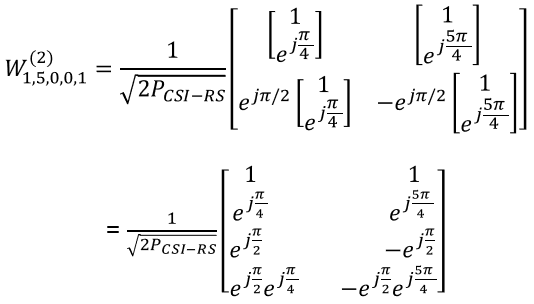Putting All these together

As I mentioned, it is very challenging to understand the detailed mechanism of CSR-RS in terms of each separate component (like CSI-RS signal generation, Codebook Configuration, related RRC parameters), but still some overall flow that put all these together has been mystery to me for a long time.

Just recently with a lot of TDoc reading and a critical help from one of the active readers of my notes, I think I reached much closer to the understandings on the overall process.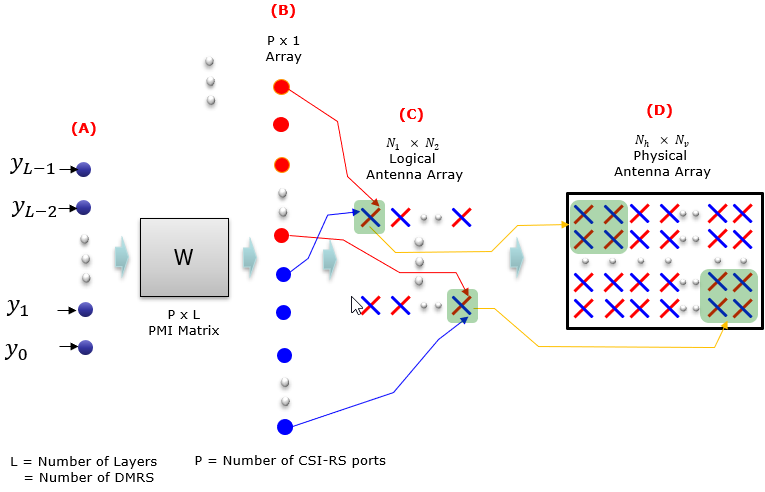Followings short notes for each process.

A -> B : This is the process of the raw data (data with DMRS) to CRS-RS antenna port. This mapping is done by multiplying the data with PMI matrix(codebook). The number of columns of this matrix is same as the number of layers (i.e, the number of DMRS antenna ports) and the number of raws of this matrix is same as the number of CSI-RS antenna ports.  This mapping can be illustrated as follows.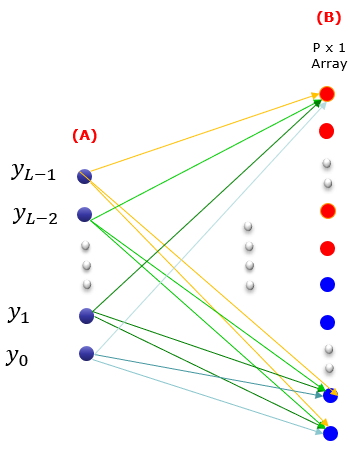B -> C : This is the process of mapping each of CSI-RS antenna port to an 1D or 2D array of cross-polarized virtual antenna ports.

C -> D : This is the process of mapping each of antenna elements in the array to a physical antenna array. The array size (the total number of elements in the array) of (D) should be equal to or greater than the size of (C). In case of beam forming, the size of (D) would be much greater than the size of (C). In this example, one polarized antenna in (C) is mapped to four polarized antenna in (D), but this mapping is just an example and the mapping would very depending on vendor. .

Does UE knows all these detailed mapping information ?

In short, UE knows the details of (A) -> (B) -> (C) via DCI and RRC  configuration, but it doesn't know anything about (D). But this technology is designed in such a way that UE does not need to know about (D). We say this as '(C) -> (D) mapping is transparent to UE'.

PortIndexFor8Ranks ::= CHOICE {

portIndex8    SEQUENCE{

rank1-8         PortIndex8 OPTIONAL, -- Need R

rank2-8         SEQUENCE(SIZE(2)) OF PortIndex8 OPTIONAL, -- Need R

rank3-8         SEQUENCE(SIZE(3)) OF PortIndex8 OPTIONAL, -- Need R

rank4-8         SEQUENCE(SIZE(4)) OF PortIndex8 OPTIONAL, -- Need R

rank5-8         SEQUENCE(SIZE(5)) OF PortIndex8 OPTIONAL, -- Need R

rank6-8         SEQUENCE(SIZE(6)) OF PortIndex8 OPTIONAL, -- Need R

rank7-8         SEQUENCE(SIZE(7)) OF PortIndex8 OPTIONAL, -- Need R

rank8-8         SEQUENCE(SIZE(8)) OF PortIndex8 OPTIONAL -- Need R

},

portIndex4    SEQUENCE{

rank1-4         PortIndex4 OPTIONAL, -- Need R

rank2-4         SEQUENCE(SIZE(2)) OF PortIndex4 OPTIONAL, -- Need R

rank3-4         SEQUENCE(SIZE(3)) OF PortIndex4 OPTIONAL, -- Need R

rank4-4         SEQUENCE(SIZE(4)) OF PortIndex4 OPTIONAL -- Need R

},

portIndex2    SEQUENCE{

rank1-2         PortIndex2 OPTIONAL, -- Need R

rank2-2         SEQUENCE(SIZE(2)) OF PortIndex2 OPTIONAL -- Need R

},

portIndex1         NULL

}

CodebookConfig ::= SEQUENCE {

codebookType CHOICE {

type1 SEQUENCE {

subType CHOICE {

typeI-SinglePanel SEQUENCE {

nrOfAntennaPorts CHOICE {

two SEQUENCE {

twoTX-CodebookSubsetRestriction BIT STRING (SIZE (6))

},

moreThanTwo SEQUENCE {

two-one-TypeI-SinglePanel-Restriction        BIT STRING (SIZE (8)),

two-two-TypeI-SinglePanel-Restriction        BIT STRING (SIZE (64)),

four-one-TypeI-SinglePanel-Restriction       BIT STRING (SIZE (16)),

three-two-TypeI-SinglePanel-Restriction      BIT STRING (SIZE (96)),

six-one-TypeI-SinglePanel-Restriction        BIT STRING (SIZE (24)),

four-two-TypeI-SinglePanel-Restriction       BIT STRING (SIZE (128)),

eight-one-TypeI-SinglePanel-Restriction      BIT STRING (SIZE (32)),

four-three-TypeI-SinglePanel-Restriction     BIT STRING (SIZE (192)),

six-two-TypeI-SinglePanel-Restriction        BIT STRING (SIZE (192)),

twelve-one-TypeI-SinglePanel-Restriction     BIT STRING (SIZE (48)),

four-four-TypeI-SinglePanel-Restriction      BIT STRING (SIZE (256)),

eight-two-TypeI-SinglePanel-Restriction      BIT STRING (SIZE (256)),

sixteen-one-TypeI-SinglePanel-Restriction    BIT STRING (SIZE (64))

},

typeI-SinglePanel-codebookSubsetRestriction-i2     BIT STRING (SIZE (16))

}

},

typeI-SinglePanel-ri-Restriction BIT STRING (SIZE (8))

},

typeI-MultiPanel SEQUENCE {

two-two-one-TypeI-MultiPanel-Restriction          BIT STRING (SIZE (8)),

two-four-one-TypeI-MultiPanel-Restriction         BIT STRING (SIZE (16)),

four-two-one-TypeI-MultiPanel-Restriction         BIT STRING (SIZE (8)),

two-two-two-TypeI-MultiPanel-Restriction          BIT STRING (SIZE (64)),

two-eight-one-TypeI-MultiPanel-Restriction        BIT STRING (SIZE (32)),

four-four-one-TypeI-MultiPanel-Restriction        BIT STRING (SIZE (16)),

two-four-two-TypeI-MultiPanel-Restriction         BIT STRING (SIZE (128)),

four-two-two-TypeI-MultiPanel-Restriction         BIT STRING (SIZE (64))

},

ri-Restriction BIT STRING (SIZE (4))

}

},

codebookMode INTEGER (1..2)

},

type2 SEQUENCE {

subType CHOICE {

typeII SEQUENCE {

n1-n2-codebookSubsetRestriction CHOICE {

two-one               BIT STRING (SIZE (16)),

two-two               BIT STRING (SIZE (43)),

four-one              BIT STRING (SIZE (32)),

three-two             BIT STRING (SIZE (59)),

six-one               BIT STRING (SIZE (48)),

four-two              BIT STRING (SIZE (75)),

eight-one             BIT STRING (SIZE (64)),

four-three            BIT STRING (SIZE (107)),

six-two               BIT STRING (SIZE (107)),

twelve-one            BIT STRING (SIZE (96)),

four-four             BIT STRING (SIZE (139)),

eight-two             BIT STRING (SIZE (139)),

sixteen-one           BIT STRING (SIZE (128))

},

typeII-RI-Restriction    BIT STRING (SIZE (2))

},

typeII-PortSelection SEQUENCE {

portSelectionSamplingSize              ENUMERATED {n1, n2, n3, n4} OPTIONAL,

typeII-PortSelectionRI-Restriction     BIT STRING (SIZE (2))

}

},

phaseAlphabetSize                            ENUMERATED {n4, n8},

subbandAmplitude                             BOOLEAN,

numberOfBeams                                ENUMERATED {two, three, four}

}

}

}

Reference

 3GPP TSG-RAN WG1 #87  R1-1612661 : Advanced CSI Codebook Structure

3GPP TSG-RAN WG1 #86  R1-166579 : Discussion on codebook design to support up to 32 ports CSI RS

3GPP TSG-RAN WG1 #86  R1-167140 : Codebook for up to 32 ports CSI-RS

3GPP TSG-RAN WG1 #86  R1-166342 : Codebook Design for Class A CSI Reporting up to 32 Ports

3GPP TSG-RAN WG1 #86  R1-166445 : Codebook design for {20, 24, 28, 32} ports

 3GPP TSG-RAN WG1 #90 R1-171nnnn [DRAFT] Summary on views on CSI reporting for Type I and Type II

 3GPP TSG RAN WG1 Meeting #82b   R1-155490  Rank 1-2 codebook for Class A CSI reporting

 3GPP TSG RAN WG1 Meeting #85 : R1-164776  View on class A codebook extension

 3GPP TSG RAN WG1 Meeting #85 : R1-164777  Hybrid PMI codebook based CSI reporting and simulation results

 3GPP TSG RAN WG1 Meeting #85 : R1-164780 Linear combination (LC) codebook based CSI reporting and simulation results

 3GPP TSG RAN WG1 Meeting #85 : R1-164857 Discussion on codebook design to support up to 32 ports CSI-RS

 3GPP TSG-RAN WG1#85  : R1-165097 Class A Codebook Design for up to 32 Ports in Rel-14

3GPP TSG RAN WG1 Meeting #83 : R1-156790 Rank 2 codebook -- summary of RAN1 proposals and performance

3GPP TSG RAN WG1 Meeting #83 : R1-156791 Rank 3-8 codebook -- summary of RAN1 proposals and performance

3GPP TSG RAN WG1 Meeting #83 : R1-156831 Codebook design for Class B

3GPP TSG RAN WG1 Meeting #83 : R1-157003 16 port codebook design and evaluations

3GPP TSG RAN WG1 Meeting #83 : R1-157164 Codebook design for elevation BF and FD-MIMO

3GPP TSG-RAN WG1#83          : R1-157208 Class A Codebook Design for Rank 1-2

3GPP TSG-RAN WG1#83          : R1-157209 Class A Codebook Design for Rank 3-8

3GPP TSG RAN WG1 Meeting #83 : R1-157300 Codebook Design for rank 2 for 2D antenna arrays

3GPP TSG RAN WG1 Meeting #83 : R1-157301 Codebook Design for rank 3 to 8 for 2D antenna arrays

3GPP TSG RAN WG1 Meeting #83 : R1-157427 Samsung, Ericsson, NTT DOCOMO, CATT